# QR DecompositionSuppose we have a problem that can be modeled by the system of equations Ax = b with a matrix A and a vector b. We have already shown how to use Gaussian Elimination to solve these methods, but I would like to introduce you to another method – QR decomposition. In addition to solving the general system of equations, this method can also be used in a number of other algorithms including the linear regression analysis to solve the least squares problem and to find the eigenvalues of a matrix.

Generally, when we see a system of equations, the best way to proceed in solving it is Gaussian elimination, or LU decomposition. However, there are some very special matrices where this method can lead to rounding errors. In such cases, we need a better, or more stable algorithm, which is when the QR decomposition method becomes important.

Any matrix A, of dimensions m by n with m >= n, can be represented as the product of two matrices, an m by m orthogonal matrix Q, i.e. QTQ = I, and an m by n upper triangular matrix R with the form [R 0]T. We perform this decomposition by will first converting the columns of the matrix A into vectors. Then, for each vector ak, we will calculate the vectors uk and ek given by

uk =i = 1 to k-1projej ak
and
ek = uk / ||uk||
Then Q = [e1, …, en] and

R =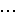00 0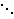# The Gram-Schmidt Process and Orthogonal Vectors

Suppose I gave you some red fingerpaint and asked you to make all the colors you could from this paint. You’d probably come up with a diverse collection of pinks, reds and burgendys – going through the range of reds – but you would be unable to produce a color that does not depend solely on red, like purple.If I were to ask you to produce purple, your reply may be something like “well, give me blue and I’ll be able to color in purple”. When we think in terms of colors, it is easy to understand the concept of the span of a set of colors. In this context, span refers to the set of colors we can create from our original colors.

Now, lets think in terms of numbers (actually vectors) instead of colors. If I gave you a similar task as above, but instead of the color red, I gave you the vector (1, 0, 0) and told you to see what other numbers you could get from this (by scalar multiplication and the addition of any two vectors already produced) then you would probably come back to me and show me how you could use the vector (1, 0, 0) to produce the entire real number line in that first dimension, but leaving the other coordinates at 0.

If (similar to the color example) I asked you to use the vector (1, 0, 0) to produce the vector (1, 1, 0), then you may reply with a similar statement as above: “you give me the vector (0, 1, 0) and I’ll produce (1, 1, 0)”. That’s because the vectors (1, 0, 0) and (1, 1, 0) are linearly independent. This is a mathematical way of saying what we’ve already stated, that you cannot get one vector as a scalar multiple of the other vector for any real number scalar.

If two vectors are linearly independent then neither one belongs to the span of the other. Just as you cannot create blue from red, you cannot create red from blue. So this means that if I were to give you the colors red and blue, then the set of colors that you can create has increased from what you could create from either only red or only blue. Similarly, the vector sets {(1, 0, 0), (0, 1, 0)} spans more vectors than just {(1, 0, 0)} or {(0, 1, 0)}.

Consider then the following two sets of vectors: {(1, 0, 0), (0, 1, 0)} and {(1, 0, 0), (1, 1, 0)}. Notice that (1, 1, 0) [belongs to] span({(1, 0, 0), (0, 1, 0)}) because (1, 1, 0) = (1, 0, 0) + (0, 1, 0). Likewise (0, 1, 0) [belongs to] span({(1, 0, 0), (1, 1, 0) because (0, 1, 0) = (1, 1, 0) – (1, 0, 0). So we can see that these two sets span the same sets of vectors. But which is a better set to use?

Lets to back to colors and think of the set {red, purple}. Here we can think of purple as a simple combination of red and blue (i.e. purple = red + blue). What happens if we mix red and purple? If we think of it in terms of an axis, the fact that purple contains red in it means that as we walk along the purple axis, we are walking along the red axis as well. If we considered the set {red, blue}, we see that this is not happening. As we change the amount of red in our color, the amount of blue is unaffected. Likewise, if we change the amount of blue, the amount of red is not affected. If we were to draw these axes, we could see that this happens because the colors red and blue are perpendicular (or orthogonal) to one another, while red and purple are not.Replacing these colors with vectors again, we get the same thing. We have the option of using the vector set {(1, 0, 0), (1, 1, 0)} or {(1, 0, 0), (0, 1, 0)} as our axis and it is better to use the second set because the two vectors are not just independent of one another, but also are at a 90[degree] angle, or are orthogonal to one another.

When given a set of vectors (or a set of colors), an important problem is to determine the span of those vectors and to be able to produce an orthogonal set of vectors that spans that same space. The Gram-Schmidt process provides a procedure for producing these orthogonal vectors.

The way the procedure works is to build an orthogonal set of vectors from the original set by computing the projection of the current vector being worked on in terms of the previous vectors in the orthogonal set. This projection procedure is defined as proju(v) = (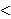u, v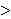/u, u)u. The formula for the ith vector of the Gram-Schmidt process is

ui = vi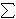j = 1 to i-1 projuj(vi).

Here is a script I’ve written to help with this process.